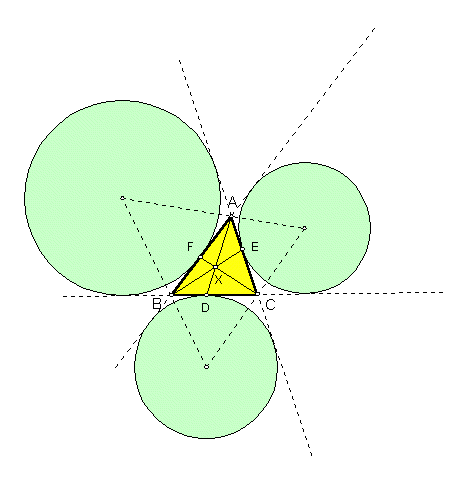# NAGEL POINT

 In the plane of any triangle ABC, let Ea = the A-excircle, Eb = the B-excircle, Ec = the C-excircle. Let D = the point where Ea touches side BC, E = the point where Eb touches side CA, F = the point where Ec touches side AB. The lines AD, BE, CF meet in a point, labeled X in the figure. It is called the Nagel point of triangle ABC.Another construction of the Nagel point follows. Let

d = the point half way around the perimeter of ABC, starting at A,
e = the point half way around the perimeter of ABC, starting at B,
f = the point half way around the perimeter of ABC, starting at C,

The lines Ad, Be, Cf meet in the Nagel point.